# himaginary's blog

## 2011年6月12日日曜日

### Analogy between Gibbs free energy and excess demand for money

In thermal physics, Gibbs free energy is defined as follows:
G ≡ U - τσ + pV

where U is the internal energy, τ is the temperature, σ is the entropy, p is pressure, V is volume.

Therefore,
dG = dU - τdσ - σdτ + pdV + Vdp

Now: "Consider a system in thermal contact with a heat reservoir R1 at temperature τ and in mechanical contact with a pressure reservoir R2 that maintains the pressure p, but cannot exchange heat." (Kittel p.262)

In this system S, dτ=0 and dp=0, so
dGS ＝ dUS - τdσS + pdVS

In equilibrium, this equals zero for each set of (τ,p).

In monetary economics, excess demand for money can be derived from equation of exchange as follows:
G ≡ PY - MV

where P is the price, Y is the output, M is the money, V is the velocity of money.

Therefore,
dG = dPY + PdY - dMV - MdV

Now, consider a mediating system in financial contact with a central bank R1 that maintains the money M and in transactional contact with a real economy R2 that maintains the output Y.

In this system S, dM=0 and dY=0, so
dGS ＝ YdPS - MdVS

In equilibrium, this equals zero for each set of (M,Y).This analogy is the idea behind the previous post.

From this analogy, you can derive the monetary economics version of Clausius-Clapeyron relation. Note that in this analogy p(pressure) corresponds to Y(output), V(volume) corresponds to P(price), τ(temperature) corresponds to M(money), and σ(entropy) corresponds to V(velocity of money).

## 2011年6月11日土曜日

### Phase transition analogy of inflation

When zero interest rate is not enough to revive the depressed economy, quantitative easing is the next step for monetary policy to proceed. However, it is often the case that small increase in money supply is not enough. You'll need large increase in money supply to obtain some effect, but there is always risk of doing too much. That's what the ketchup theory of inflation warns us.

It may be interesting to think about this by analogy to latent heat in phase transition. Latent heat is what substance needs to complete phase transition (eg. from ice to water, liquid to gas). If the heat added is less than the latent heat, phase transition remains incomplete. So, in terms of quantitative easing, latent heat corresponds to the certain amount of money which is needed to change the state of economy.

Speaking of phase transition, it may be also interesting to draw phase diagram in monetary policy. If you compare liquidity-trap economy to solid phase, normal economy to liquid phase, and hyperinflation economy to gas phase, it goes like this: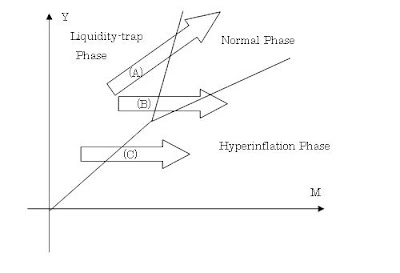Here I took money supply M as X-axis(temperature in actual phase transition), and real output Y as Y-axis(pressure in actual phase transition).

Arrow (A) is what quantitative easing aims at. That is, by the appropriate increase in money, economy makes transition from liquidity-trap phase to normal phase.

However, if you do too much, the economy may jump over normal phase and move into hyperinflation phase(arrow (B)).
Or, real economy may not be strong enough to move back to normal state in the first place, and economy may move directly into hyperinflation phase(arrow (C)).
These scenarios are what inflationistas worry about.

BTW, I've also done some maths on this analogy, and will explain them in later post(s).

## 2010年11月28日日曜日

### The Difference between Quantitative Easing and changing the treasury maturity structure

Everyone says Quantitative Easing and changing the treasury maturity structure are equivalent (e.g. Paul Krugman, James Hamilton, Robert Waldmann, Robert Hall, Robert Barro).

However, if you look at each bond market through surplus analysis, you can see the difference between the two operations.

A. Changing the treasury maturity structure

A-1. Long-Term Bond Market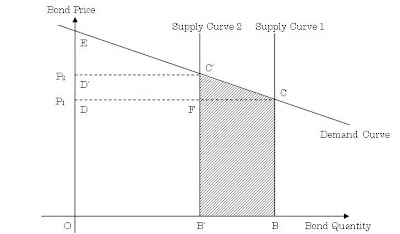When the government reduces the issuance of long-term treasury bond, the supply curve shifts from "Supply Curve 1" to "Supply Curve 2". As a result, total surplus declines (shaded area). The government surplus changes from OBCD to OB'C'D'. Bond buyers' surplus changes from DCE to D'C'E.

(Here I assumed that the government doesn't change the amount of issuance according to bid-price, so that the supply curve is vertical. The government may have the reserve price under which it cancels the issuance, but here I assume that the reserve price is zero for simplicity [Although this is unrealistic assumption, it doesn't affect the main observation of this post]. In that case, the government surplus equals the amount of issuance.)

Of the bond buyers' surplus decrease, DFC'D' transfers to the government. The other part of the decrease, FCC', is the deadweight loss.

A-2. Short-Term Bond MarketThe government wants to offset the above reduction in the revenue it raises from the long-term bond market by increasing the short-term bond issuance. So, the supply curve shifts from "Supply Curve 1" to "Supply Curve 2" in the short-term bond market.

On the other hand, as short-term interest rate has hit the zero bound, the demand curve is flat. That is, T-bill and the money is equivalent in this market (That is the point many commentators - such as Krugman - emphasize). So, the bond buyers' surplus doesn't change before and after the issuance increase.

As a result, only the government's surplus changes to compensate the reduction it suffered in the long-term bond market (shaded area).

B. Quantitative Easing

Long-Term Bond Market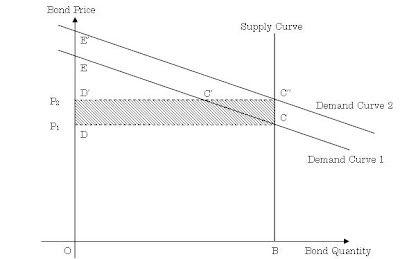In Quantitative easing, the Central Bank participates in the purchase of the long-term bond. So the demand curve shifts from "Demand Curve 1" to "Demand Curve 2". In that case, the bond buyers' surplus doesn't change; it just shift from DCE to D'C''E' (*1). On the other hand, the government's surplus increases by the shaded area DCC''D', which equals ECC''E'.

That government's surplus increase is the amount of "seigniorage" which the Central Bank monetized. It shows up explicitly in the change in bond price as shown in the above figure, so its effect on inflation expectation is more "direct" than in changing the treasury maturity structure case.

(When the Central Bank doesn't purchase the bond directly from the government, some of the surplus is diverted to the arbitrage profit of the bank. But if the competition among banks works well, that arbitrage profit approaches zero in the limit.)

(*1) Of the bond buyers' surplus after the shift D'C''E', C'C''E'E is the surplus of the Central Bank. So the private buyers' surplus is D'C'E, which equals to the bond buyers' surplus when the bond supply was decreased (A-1). However, there is no deadweight loss this time.

In sum, changing the treasury maturity structure ends up in the decrease of the long-term bond buyers' surplus with deadweight loss. In contrast, QE increases the government's surplus while keeping the total bond buyers' surplus unchanged. (Although the private bond buyers' surplus decreases in the latter case as much as the former case, there is no deadweight loss.)

Some may say that decrease in the issuance of long-term treasury bonds, along with decrease in the surplus of their buyers, is not such a big deal. But didn't Ricardo Caballero and Brad Delong emphasize about the current shortage in safe assets? Decreasing the issuance of long-term treasury bonds exacerbates that shortage.

While the Central Bank's purchase of the bonds seems to be equivalent to the decrease in the issuance of the bonds on the consolidated government basis, total outstanding of the bonds is different in each case nonetheless. Thinner total outstanding makes the market more vulnerable to the demand shock, which is likely to occur considering the craving for safe assets Caballero and Delong noted.

Besides, that difference in total outstanding involves deadweight loss as shown above.

## 2010年4月9日金曜日

### A hyperinflation model: circumventing Buiter's critique by holding nominal money growth constant

In hyperinflation model, Cagan money demand function is commonly used (See David Romer, "Advanced Macroeconomics").

m = C・exp(-bπ)

where m is real money stock, π is the rate of inflation, b and C are constant parameters.

Rewriting this as an equation for inflation gives us

π = (-1/b)・ln(m/C)

So, government seigniorage d can be written as

d = Δm + πm
= Δm - (m/b)・ln(m/C)

Therefore,

Δm = d + (m/b)・ln(m/C)

Differentiating this with m gives us

∂Δm/∂m = (1/b)・{ln(m/C) + 1}

Which becomes zero when m = C・exp(-1).

Second derivative of Δm is

∂2Δm/∂m2 = 1/bm

which is strictly positive. So

Δm = d - (C/b)・exp(-1)

is the minimum value of Δm.

Here, d = (C/b)・exp(-1) gives us the maximum value of d presuming Δm=0.

Proof)
∂(πm)/∂π = C・exp(-bπ) - bCπ・exp(-bπ)
= (1-bπ)・C・exp(-bπ)
Therefore, πm, or seigniorage when Δm=0,
becomes maximum when π = 1/b.
At that point,
m = C・exp(-1)
and
d = (C/b)・exp(-1)

So, if we denote this maximum d under stationary condition as d*, the above Δm minimum value becomes

Δm = d - d*

Hyperinflation is thought to happen when d > d*. However, in that case, Δm becomes positive. Which means, m increases, and π＝(-1/b)・ln(m/C) decreases. This situation should be called hyperdeflation, not hyperinflation. This is the paradox noted by Willem Buiter (for detail, see Alexandre Sokic, "Monetary hyperinflation, speculative hyperinflation and modelling the use of money").

And if we think about nominal money growth rate (let's denote this gM) in this hyperdeflation case, it can be shown that

ΔgM / gM = - (Δm / m)

This is because d is assumed to be constant. So, if Δm is positive, ΔgM is negative. In this paradox case, nominal money growth decreases, but as inflation decreases further, real money growth becomes positive.

If you describe people's behavior in this case, it's something like this:
They watch government move and say, "Oh dear, the government is trying to obtain seigniorage beyond possible point. Let's suppress our economic activity and bring inflation down, so that real money stock increases and the government can obtain and maintain that seigiorage. Then, the central bank doesn't need to print more money. It can even print less money."

Obviously, this just cannot happen -- at least, not in the secular world.

In the real world, gM is the only political tool. So, if the government wants more seigniorage, it makes the central bank to increase gM. At any rate, it never lets gM decrease.

Now, let's see what happens if we hold gM constant.

If gM is constant, Δm becomes

Δm = gMm + (m/b)・ln(m/C)

Differentiating this with m gives us

∂Δm/∂m = gM + (1/b)・{ln(m/C) + 1}

Which becomes zero when m = C・exp(-1-bgM).

Second derivative of Δm is

∂2Δm/∂m2 = 1/bm

which is strictly positive. So

Δm = -(C/b)・exp(-1-bgM)

is minimum value of Δm. And this is strictly negative, which means hyperinflation can occur.

And, if gM is constant, we can obtain general solution for π, which is
π = gM + A・exp(t/b)
where A is constant and t denotes time.

Proof)
Cagan money function
m = C・exp(-bπ)
gives us
(dt/dm)/m = -b(dπ/dt)
And seigniorage is
d = Δm + πm = gMm
So, the following differential equation holds
(Note that I use Δm and dm/dt interchangeably).
gM = -b(dπ/dt) + π
Solving this equation gives the above solution.

As noted above, the government would like to increase gM. This constant-gM-solution may be fit for some short period Δt, but it's unlikely to be adequate description for longer period.

However, maybe increasing gM stepwise in this solution can serve as the ad-hoc but tolerable way to describe the hyperinflation. The following graphs are from an example of such a simulation (click to enlarge).(d=left-hand-axis, gM=right-hand-axis)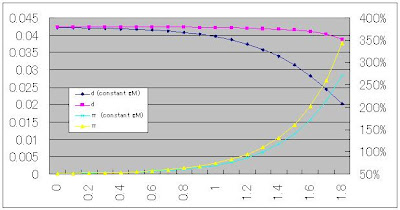(d=left-hand-axis, π=right-hand-axis)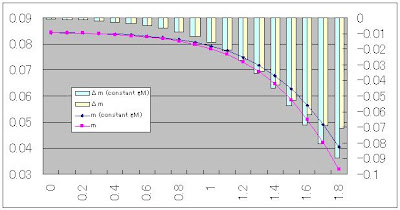(m=left-hand-axis, Δm=right-hand-axis)

In this simulation, I set b=1/3, C=0.1, Δt=0.1, and gM(initial value)=0.5. Hence, initial d=gM・C・exp(-bgM)=0.0423.
"Constant gM" case is where gM is always 0.5.
In not-constant-gM-case, I increased gM each period so that d becomes approximately constant.

## 2009年10月25日日曜日

### Incorporating NGDP into Taylor rule

Glenn Rudebusch of San Francisco FRB has proposed the following Taylor rule deduced by regression analysis.

FF Rate = 2.07 + 1.28 x Inflation - 1.95 x (Unemployment rate - NAIRU)

Paul Krugman preferred this equation to the original Taylor rule.
The original one can be expressed as follows, according to Taylor's own expression in his Bloomberg column.

FF Rate = 1 + 1.5 x Inflation + 0.5 x GDP gap

There are two merits in Rudebusch version over the original version:
1. Parameters are deduced from actual data, whereas parameters in the original version are rather ad-hoc.

2. Unemployment rate is more straightforward statistics compared to GDP gap.

On this Taylor rule matter, a commenter of this post at "Macro and Other Market Musings" blog made an interesting suggestion.

If you look at the Taylor rule, it does a balancing act between real GDP growth and inflation and only indirectly controls nominal GDP growth. It should be easy to modify the Taylor rule so it would directly control nominal GDP growth by dropping the inflation term and letting the (Yt-Yt*) term represent measured and desired GDPn instead of GDPr. The coefficients would have to be adjusted also.

It would be interesting to compare this modified Taylor rule with the standard Taylor rule over the last few years. Would this modified TR have instructed the Fed to drop the FFR at a faster rate than the standard TR over the past couple of years?

Intrigued by this comment, I defined NGDP-version Taylor rule as follows:

FF Rate = NGDP Growth Rate - 2 x (Unemployment rate - NAIRU)

In this equation, I just replaced constant term and inflation term of Rudebusch version with NGDP Growth Rate, and rounded the coefficient of Unemployment term to nearest integer. It may be crude, but with the merit of simplicity. This equation says that the target rate should be set at minimum rate that suffices dynamic efficiency, once NAIRU is accomplished.

Here is the graph of rate calculated by this version along with Rudebusch version and actual rate: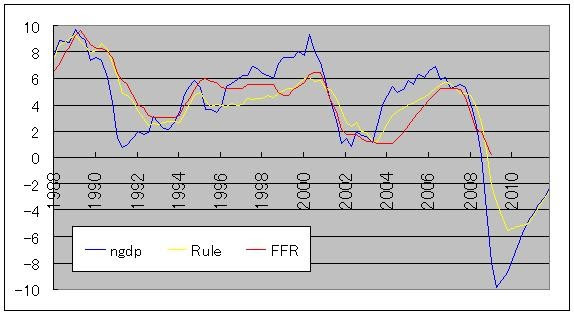"FFR" is the actual Federal Fund Rate, "Rule" is the rate calculated by Rudebusch version, "ngdp" is the rate by NGDP version.
As you can see, NGDP version does urge FRB to act more quickly and boldly than either actual FFR or Rudebusch version. And that signal is not limited to past several years. S&L crisis, IT bubble and its burst, housing bubble and its burst,... In every case, NGDP version urged FRB to hike or drop FF Rate more quickly and boldly.

But aren't the parameters of this NGDP-version Taylor rule also ad-hoc? Um, maybe. So, now, let's see what happens if we conduct regression analysis like Rudebusch did, just replacing inflation term in his version with NGDP Growth Rate term. Here is the result:

FF Rate = 3.951 + 0.214 x NGDP Growth Rate - 1.714 x (Unemployment rate - NAIRU)

Unfortunately, adjusted R-squared is not so high... 0.489, as opposed to 0.810 of Rudebusch version. The t-values are not so impressive either... 5.1, 1.6, -6.9, in above order. The t-value of NGDP term is not significant even at 10% level. This result verifies that FRB hasn't considered NGDP Growth Rate as policy target so far. (Maybe it's going to change hereafter, or, so it is hoped.)

Add this NGDP regression version to the above graph, and you get this: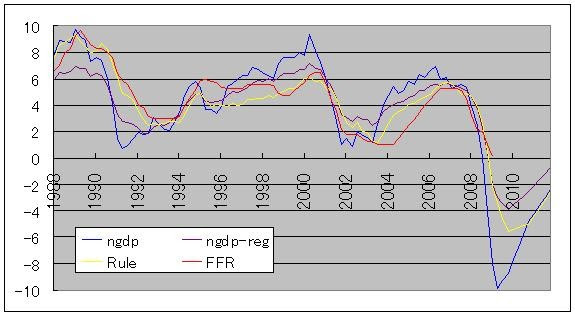You can see that the movement is rather modified than original NGDP version. However, during 2002-2003 period, this version is the highest among the four.

In fact, if you compare this to what John Taylor asserts FF Rate should have been during this period, it's very close.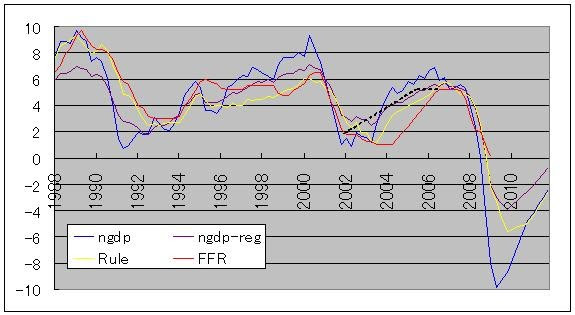The black chain line is what John Taylor asserts as derived from his original version of Taylor rule.

And as for forecast period, i.e. after 2009 Q3, NGDP-Regression version indicates the highest level of FF Rate among three. This also fits what John Taylor asserts in the forementioned Bloomberg column. That is, he asserts that today's ideal FF Rate level is not far from zero, while Krugman and other people says it's lower than -5%. Although NGDP-Regression level is around -4%, it's still nearer to zero than other two rates.

Note: As for data other than NGDP in forecast period, I used Rudebusch's data. NGDP Growth rate in forecast period is set as 2009Q4=0.6%, 2010Q4=3.7%, 2011Q4=4.1% using CBO forecast, and linearly interpolated for intermediate period.

Update(Dec 10, 2009):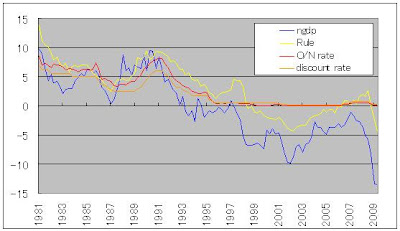(click to enlarge)

This is what it looks like in Japan. Here, too, NGDP version urges to act more quickly and boldly than either actual policy rate or Rudebusch version.

(I didn't calculate NGDP-Regression version, because that regression is almost meaningless in Japan, which has been caught in liquidity trap for almost 15 years (as you can see in the above graph). I used 3.5% as NAIRU, based on Mr. kuma_asset's estimate. O/N rate became monetary policy tool since 1995 in Japan. Until then, discount rate was the policy rate.)

## 2009年5月2日土曜日

### Paul Krugman's elementary mistakes on economics

In my previous post, I showed that the story of export-led recovery of mid-00's Japan is a myth.

However, there are still many economists who believe that myth. Paul Krugman may be the most famous one among them.

For example, in his April 27 blog post, he showed the following graph.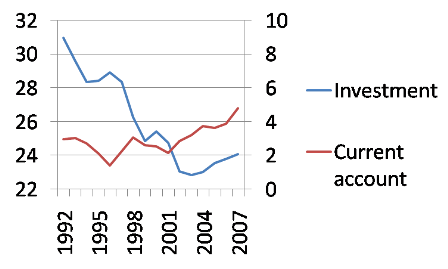And he writes,

Here’s Japanese investment and current account balance (trade balance broadly defined), both as a share of GDP, over the period 1992-2007:
Investment was actually lower, as a share of GDP, in 2007 than it had been in 2000. What had changed was the trade balance.

• Mistake No.1

• 　　What he used as investment is actually Gross Fixed Capital Formation (GFCF).
And by definition, it contains Government Investment.

• Mistake No.2

• 　　He failed to recognize that Current account balance and trade balance could be very different.

Let's start with investment. Below is the graph of GFCF and Private Investment (% of GDP).(source: Cabinet Office HP; click to enlarge)

You can see that while GFCF stagnated after 2003 as Krugman said, Private Investment did increase.

Why this discrepancy occurred? As GFCF consists of Private Investment, Residential Investment, and Public Investment, let's see the movement of each of the component.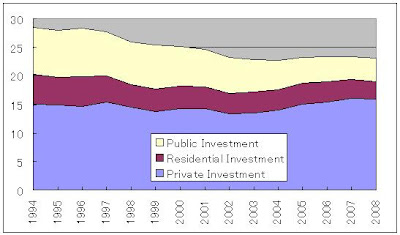Private Investment increased, but the cutback of Public Investment more than offset that increase. The cutback was done because the economy recovered, and budget deficit cannot go on forever. So using GFCF as the sign of lack of investment is total nonsense.

And here is current account and trade balance graph (% of GDP).Both moved in parallel until 2000, but after that, the movement became very different. Trade balance actually shrank from 2004 till 2006.

The reason of this discrepancy can be also understood by seeing the movement of components.Income from abroad increased, and that increase was main cause of the discrepancy between current account balance and trade balance.

As a matter of fact, this is not the first time Krugman made mistake on Japanese basic economic statistics. Below is the graph he used in his February 28 post and April 2 post.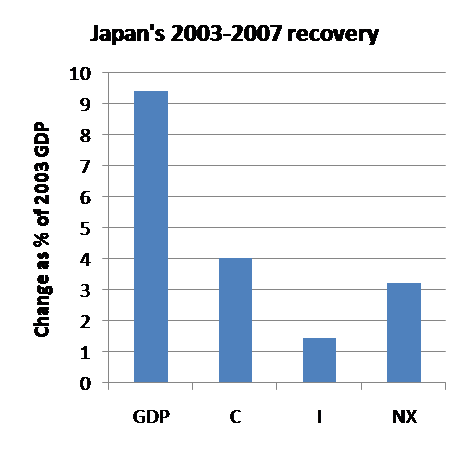And here is the corresponding graph drawn using Japanese SNA statistics. Nominal basis growth is also shown for comparison.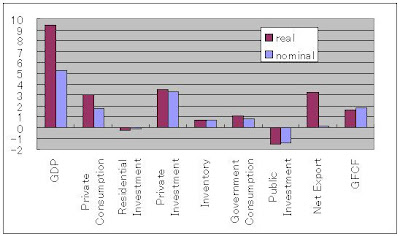You can see that Krugman is using GFCF as "I" in the above graph, too. And you can see "NX" contribution is next to nothing on nominal basis.

I repeatedly pointed out these facts in his blog comment, but apparently it didn't get through to him.

### Was recovery of Japanese economy in mid-00's led by export?

No, it wasn't. And here is the reason.This graph shows that net export did contribute to nominal GDP growth in 2002-2003, but after that, the contribution was marginal. In 2004-2005, the contribution was even negative.

But what is important is real GDP, and net export contributed to that growth, right?

...Um, not necessarily.

Well, it's true that net export contribute to real GDP growth, as I showed in the last graph in my previous post. I'll show the graph again.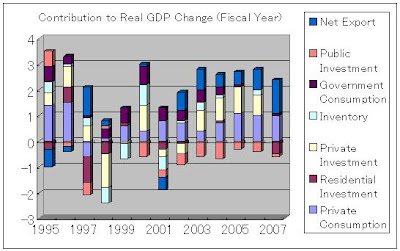This is in stark contrast to the nominal-based graph.

Then, what caused this difference?

Two graphs below answer that question.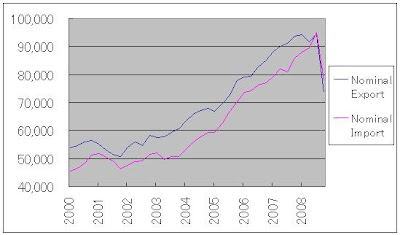(quarterly basis, seasonally adjusted annual rate, billion yen)

On real basis, import did not increase much from 2003 onward, but export did.
On the other hand, both import and export increased on nominal basis, so net export did not change much.

This means that import price hike, caused by the price hike of oil and other natural resources, was what led to contribution of net export to GDP growth on real basis. Of course, it has nothing to do with the increase in export. Yes, export did increase, but that increase was consumed by the increase in import.

In other words, Japan increased export to pay for the hike in price what they import. Otherwise it would have run trade deficit. That situation could be hardly called export-led growth.

Put another way, real GDP growth was somewhat due to net export, but real GDI (Gross Domestic Income) growth wasn't. This is because real GDI is approximately calculated by replacing net export factor from real to nominal. From this point of view, Japan is in contrast to Canada as Prof. Stephen Gordon explained.

## 自己紹介

This blog is some thoughts on economics by a Japanese non-economist. Translated from my Japanese blog.